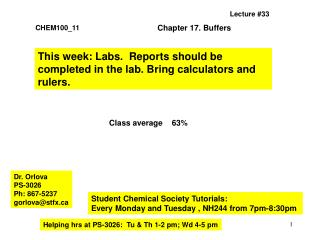DownloadDownload PresentationCHEM100_11

# CHEM100_11

Télécharger la présentation## CHEM100_11

- - - - - - - - - - - - - - - - - - - - - - - - - - - E N D - - - - - - - - - - - - - - - - - - - - - - - - - - -
##### Presentation Transcript

1. Lecture #33 Chapter 17. Buffers CHEM100_11 This week: Labs. Reports should be completed in the lab. Bring calculators and rulers. Class average 63% Dr. Orlova PS-3026 Ph: 867-5237 gorlova@stfx.ca Student Chemical Society Tutorials: Every Monday and Tuesday , NH244 from 7pm-8:30pm Helping hrs at PS-3026: Tu & Th 1-2 pm; Wd 4-5 pm

2. pH 14.0 11.0 10.0 9.0 8.0 pH=7 7.0 5.0 4.0 3.0 2.0 1.0 0.0 20mL mL NaOH • Strong Acid-Strong Base Titrations • A plot of pH versus volume of acid (or base) added is called a titration curve. • Lets add 20 mL of 0.1 M NaOH to 20mL of 0.1 M HCl. OH- Equivalence point NaCl + H2O H+ pHHCl = 1

3. You are titrating 25.0 mL of 0.1 M HNO3 solution with 0.1M KOH. HNO3 (aq) + KOH (aq)  KNO3 (aq) + H2O (l) Calculate pH when: a) 24.9 mL of 0.1 M KOH have been added (HNO3 is still in excess) b) 25.1 mL of 0.1M KOH have been added (overtitrated- KOH is in excess)

4. HNO3 (aq) + KOH (aq)  KNO3 (aq) + H2O (l) a) Moles H+ : (0.1mol/L x 0.0250 L) – (0.1mol/L x 0.0249) = 0.00001mol New volume: 0.0250L + 0.0249L = 0.0499L M H+ : 0.00001mol/ 0.0499 L = 0.0002 M pH = -log 2.0 x 10-4 =3.70

5. b) Moles OH- : (0.1mol/L x 0.0251 L) – (0.1mol/L x 0.0250) = 0.00001mol M OH- : 0.00001mol/ 0.0501 L = 0.0001996 M pOH = -log 1.996 x 10-4 =3.70 pH = 14-3.70 = 10.3 Useful information: 1mL = ca. 20 drops; 1 drop = 0.05mL In the vicinity of the equivalence point, 4 drops of 0.1 M KOH changed pH from 3.7 to 10.3

6. pH 14.0 11.0 10.0 9.0 8.0 7.0 5.0 4.0 3.0 2.0 1.0 0.0 20mL mL NaOH Titration of a weak acid with a strong base 20 mL of 0.1M solution of acetic acid is titrated with 0.1 M solution of NaOH Ka = 1.8 x 10-5

7. pH 14.0 11.0 10.0 9.0 8.0 7.0 5.0 4.0 3.0 2.0 1.0 0.0 20mL mL NaOH OH- Equivalence point pH > H+

8. pH before titration: Ka = [H+][CH3COO-]/[CH3COOH] X2/0.1 = 1.8 x 10-5 ; X2 = 1.8 x 10-6 X= √1.8 x 10-3 = 1.3 x 10-3 pH = -log 1.3 x 10-3 = 2.89

9. pH between initial and equivalent points CH3COOH (aq) + NaOH (aq)  CH3COONa(aq) + H2O (l) CH3COOH (aq) + OH- (aq)  CH3COO- (aq) + H2O (l) BUFFER! To find pH of the buffer, make 2 steps: 1. Consider neutralization rxn and using stoichiometric calculations find concentrations of CH3COOH and CH3COO- in the solution 2. Consider buffer’s equilibrium and find [H+] using reaction table or Henderson- Hasselbalch equation

10. Calculate pH when 18 mL of NaOH have been added to 20 mL of 0.1M CH3COOH (Ka = 1.8 x 10-5; pKa = 4.74) 1. Stoichiometric calculations Moles AH : 0.1 mol/L x 0.020L = 0.0020 mol Moles OH- : 0.1 mol/L x 0.018L = 0.0018 mol Before rxn 0.0020 0.0018 0 CH3COOH (aq) + OH- (aq)  CH3COO- (aq) + H2O (l) After rxn 0.0002 0 0.0018

11. But because [H+] = Ka [HA]/[A-] Ratio of molarities is the same as ratio of moles We can use moles Formally, we should use molarities Molarities: 0.0002/0.038 = 0.0053: 0.0018/0.038 =0.047 Same is valid for Henderson-Hasselbalch pH = pKa + log [CH3COO-]/[CH3COOH] pH = 4.74 + log (0.0018/0.0002) =4.74 + 0.95 = 5.69